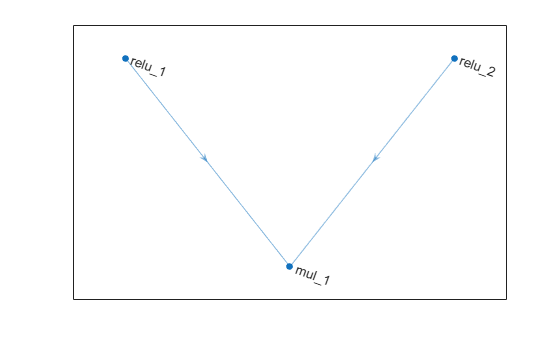# multiplicationLayer

Multiplication layer

## Description

A multiplication layer multiplies inputs from multiple neural network layers element-wise.

Specify the number of inputs to the layer when you create it. The inputs to the layer have the names `'in1','in2',...,'inN'`, where `N` is the number of inputs. Use the input names when connecting or disconnecting the layer by using `connectLayers` or `disconnectLayers`, respectively. The size of the inputs to the multiplication layer must be either same across all dimensions or same across at least one dimension with other dimensions as singleton dimensions.

## Creation

### Syntax

``layer = multiplicationLayer(numInputs)``
``layer = multiplicationLayer(numInputs,'Name',name)``

### Description

example

````layer = multiplicationLayer(numInputs)` creates a multiplication layer that multiplies `numInputs` inputs element-wise. This function also sets the `NumInputs` property.```

example

````layer = multiplicationLayer(numInputs,'Name',name)` also sets the `Name` property.```

## Properties

expand all

Number of inputs to the layer, specified as a positive integer greater than or equal to 2.

The inputs have the names `'in1','in2',...,'inN'`, where `N` is `NumInputs`. For example, if `NumInputs` is 3, then the inputs have the names `'in1','in2'`, and `'in3'`. Use the input names when connecting or disconnecting the layer using the `connectLayers` or `disconnectLayers` functions.

Layer name, specified as a character vector or a string scalar. For `Layer` array input, the `trainNetwork`, `assembleNetwork`, `layerGraph`, and `dlnetwork` functions automatically assign names to layers with name `''`.

Data Types: `char` | `string`

Input names, specified as `{'in1','in2',...,'inN'}`, where `N` is the number of inputs of the layer.

Data Types: `cell`

Number of outputs of the layer. This layer has a single output only.

Data Types: `double`

Output names of the layer. This layer has a single output only.

Data Types: `cell`

## Examples

collapse all

Create a multiplication layer with two inputs and the name `'mul_1'`.

`mul = multiplicationLayer(2,'Name','mul_1')`
```mul = MultiplicationLayer with properties: Name: 'mul_1' NumInputs: 2 InputNames: {'in1' 'in2'} Learnable Parameters No properties. State Parameters No properties. Show all properties ```

Create two ReLU layers and connect them to the multiplication layer. The multiplication layer multiplies the outputs from the ReLU layers.

```relu_1 = reluLayer('Name','relu_1'); relu_2 = reluLayer('Name','relu_2'); lgraph = layerGraph(); lgraph = addLayers(lgraph,relu_1); lgraph = addLayers(lgraph,relu_2); lgraph = addLayers(lgraph,mul); lgraph = connectLayers(lgraph,'relu_1','mul_1/in1'); lgraph = connectLayers(lgraph,'relu_2','mul_1/in2'); plot(lgraph);```## Version History

Introduced in R2020b